Home Practice
For learners and parents For teachers and schools
Textbooks
Full catalogue
Pricing SupportLog in

We think you are located in United States. Is this correct?

# Chapter 7: Euclidean geometry

• Content covered in this chapter includes revision of lines, angles and triangles. The mid-point theorem is introduced. Kites, parallelograms, rectangle, rhombus, square and trapezium are investigated.
• Solving problems and proving riders is only covered later in the year. The focus of this chapter is on introducing the special quadrilaterals and revising content from earlier grades.
• Revision of triangles should focus on similar and congruent triangles.
• Sketches are valuable and important tools. Encourage learners to draw accurate diagrams to solve problems.
• It is important to stress to learners that proportion gives no indication of actual length. It only indicates the ratio between lengths.
• Notation - emphasise to learners the importance of the correct ordering of letters, as this indicates which angles are equal and which sides are in the same proportion.

Geometry (from the Greek “geo” = earth and “metria” = measure) arose as the field of knowledge dealing with spatial relationships. Analytical geometry deals with space and shape using algebra and a coordinate system. Euclidean geometry deals with space and shape using a system of logical deductions.

Euclidean geometry was first used in surveying and is still used extensively for surveying today. Euclidean geometry is also used in architecture to design new buildings. Other uses of Euclidean geometry are in art and to determine the best packing arrangement for various types of objects.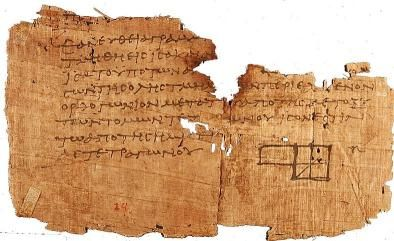A small piece of the original version of Euclid's elements. Euclid is considered to be the father of modern geometry. Euclid's elements was used for many years as the standard text for geometry.

This video highlights some of the basic concepts used in geometry.

Video: 2G5V

In Euclidean geometry we use two fundamental types of measurement: angles and distances.

GeoGebra is a useful tool to use for sketching out the worked examples and activities.

## 7.1 Introduction (EMA5M)

### Angles (EMA5N)

An angle is formed when two straight lines meet at a point, also known as a vertex. Angles are labelled with a caret on a letter, for example, $$\hat{B}$$. Angles can also be labelled according to the line segments that make up the angle, for example $$C\hat{B}A$$ or $$A\hat{B}C$$. The $$\angle$$ symbol is a short method of writing angle in geometry and is often used in phrases such as “sum of $$\angle$$s in $$\triangle$$”. Angles are measured in degrees which is denoted by $$°$$, a small circle raised above the text, similar to an exponent.

### Properties and notation (EMA5P)

In the diagram below two straight lines intersect at a point, forming the four angles $$\hat{a}$$, $$\hat{b}$$, $$\hat{c}$$ and $$\hat{d}$$.

The following table summarises the different types of angles, with examples from the figure above.

 Term Property Examples Acute angle $$0° <\text{angle} < 90°$$ $$\hat{a}$$; $$\hat{c}$$ Right angle Angle $$=90°$$ Obtuse angle $$90° < \text{angle} < 180°$$ $$\hat{b}$$; $$\hat{d}$$ Straight angle Angle $$=180°$$ $$\hat{a}+\hat{b}$$; $$\hat{b}+\hat{c}$$ Reflex angle $$180° < \text{angle} < 360°$$ $$\hat{a}+\hat{b}+\hat{c}$$ Adjacent angles Angles that share a vertex and a common side. $$\hat{a}$$ and $$\hat{d}$$; $$\hat{c}$$ and $$\hat{d}$$ Vertically opposite angles Angles opposite each other when two lines intersect. They share a vertex and are equal. $$\hat{a}=\hat{c}$$; $$\hat{b}=\hat{d}$$ Supplementary angles Two angles that add up to $$180°$$ $$\hat{a}+\hat{b}= 180°$$; $$\hat{b} + \hat{c} = 180°$$ Complementary angles Two angles that add up to $$90°$$ Revolution The sum of all angles around a point. $$\hat{a}+\hat{b}+\hat{c}+\hat{d}= 360°$$

Note that adjacent angles on a straight line are supplementary.

The following video provides a summary of the terms used to refer to angles.

Video: 2G5W

### Parallel lines and transversal lines (EMA5Q)

Two lines intersect if they cross each other at a point. For example, at a traffic intersection two or more streets intersect; the middle of the intersection is the common point between the streets.

Parallel lines are always the same distance apart and they are denoted by arrow symbols as shown below.

In writing we use two vertical lines to indicate that two lines are parallel:

$AB \parallel CD \text{ and } MN \parallel OP$

A transversal line intersects two or more parallel lines. In the diagram below, $$AB\parallel CD$$ and $$EF$$ is a transversal line.

The properties of the angles formed by these intersecting lines are summarised in the following table:

 Name of angle Definition Examples Notes Interior angles Angles that lie in between the parallel lines. $$\hat{a}$$, $$\hat{b}$$, $$\hat{c}$$ and $$\hat{d}$$ are interior angles. Interior means inside. Exterior angles Angles that lie outside the parallel lines. $$\hat{e}$$, $$\hat{f}$$, $$\hat{g}$$ and $$\hat{h}$$ are exterior angles. Exterior means outside. Corresponding angles Angles on the same side of the lines and the same side of the transversal. If the lines are parallel, the corresponding angles will be equal. $$\hat{a}$$ and $$\hat{e}$$, $$\hat{b}$$ and $$\hat{f}$$, $$\hat{c}$$ and $$\hat{g}$$, $$\hat{d}$$ and $$\hat{h}$$ are pairs of corresponding angles. $$\hat{a}=\hat{e}$$, $$\hat{b}=\hat{f}$$, $$\hat{c}=\hat{g}$$ and $$\hat{d}=\hat{h}$$.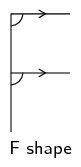Co-interior angles Angles that lie in between the lines and on the same side of the transversal. If the lines are parallel, the angles are supplementary. $$\hat{a}$$ and $$\hat{d}$$, $$\hat{b}$$ and $$\hat{c}$$ are pairs of co-interior angles. $$\hat{a}+\hat{d}= 180°$$, $$\hat{b}+\hat{c}=180°$$.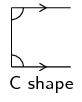Alternate interior angles Equal interior angles that lie inside the lines and on opposite sides of the transversal. If the lines are parallel, the interior angles will be equal. $$\hat{a}$$ and $$\hat{c}$$, $$\hat{b}$$ and $$\hat{d}$$ are pairs of alternate interior angles. $$\hat{a}=\hat{c}$$, $$\hat{b}=\hat{d}$$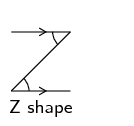This video provides a short summary of some of the angles formed by intersecting lines.

Video: 2G5X

If two lines are intersected by a transversal such that:

• corresponding angles are equal; or

• alternate interior angles are equal; or

• co-interior angles are supplementary

then the two lines are parallel.

When we refer to lines we can either write $$EF$$ to mean the line through points $$E$$ and $$F$$ or $$\overline{EF}$$ to mean the line segment from point $$E$$ to point $$F$$.

## Worked example 1: Finding angles

Find all the unknown angles. Is $$EF\parallel CG$$? Explain your answer.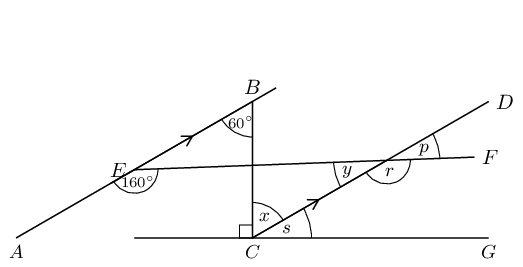### Use the properties of parallel lines to find all equal angles on the diagram

Redraw the diagram and mark all the equal angles.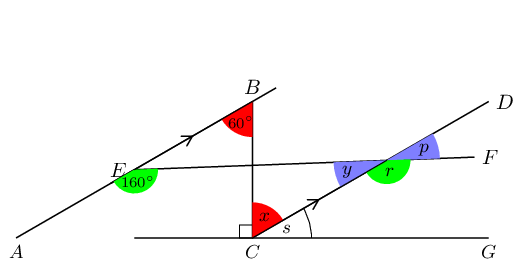### Determine the unknown angles

$\begin{array}{rll} AB& \parallel CD & \text{(given)} \\ \therefore \hat{x} & = 60° & \text{(alt }\angle \text{s; } AB \parallel CD \text{)}\\ \hat{y} + 160° & = 180° & \text{(co-int }\angle \text{s; } AB \parallel CD \text{)}\\ \therefore \hat{y} & = 20° & \\ \hat{p}& = \hat{y} & \text{(vert opp }\angle \text{s } = \text{)}\\ \therefore \hat{p}& = 20° & \\ \hat{r}& = 160° & \text{(corresp }\angle \text{s; } AB \parallel CD \text{)} \\ \hat{s} + \hat{x} + 90° & = 180° & \text{(}\angle \text{s on a str line)}\\ \hat{s} + 60° & = 90°& \\ \therefore \hat{s} & = 30° & \end{array}$

### Determine whether $$EF\parallel CG$$

If $$EF\parallel CG$$ then $$\hat{p}$$ will be equal to corresponding angle $$\hat{s}$$, but $$\hat{p}= 20°$$ and $$\hat{s}= 30°$$. Therefore $$EF$$ is not parallel to $$CG$$.

Textbook Exercise 7.1

Use adjacent, corresponding, co-interior and alternate angles to fill in all the angles labelled with letters in the diagram: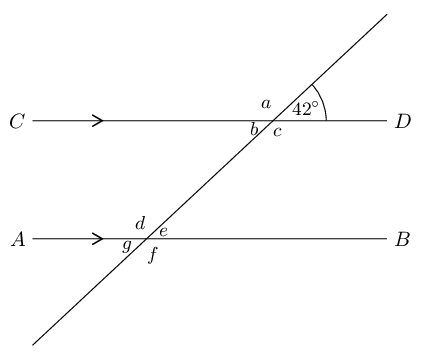You can redraw the diagram and fill in the angles as you find them.

$\begin{array}{rll} a & = 180° - 42° = 138° & \text{(}\angle \text{s on a str line)} \\ b & = 42° & \text{(vert opp } \angle\text{s } = \text{)}\\ c & = 138° & \text{(vert opp } \angle\text{s } = \text{)}\\ d & = 138° & \text{(co-int }\angle\text{s; } AB \parallel CD \text{)}\\ e & = 180° - 138° = 42° & \text{(}\angle \text{s on a str line)}\\ f & = 138° & \text{(vert opp } \angle\text{s } =\text{)}\\ g & = 42° & \text{(vert opp } \angle\text{s } =\text{)} \end{array}$

Find all the unknown angles in the figure: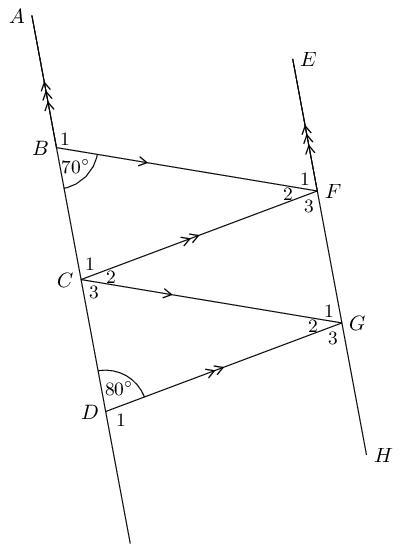$\begin{array}{rll} \hat{B}_{1} & = 180° - 70° = 110° & \text{(}\angle \text{s on a str line)} \\ \hat{D}_{1} & = 180° - 80° = 100° & \text{(}\angle \text{s on a str line)} \\ \hat{F}_{1} & = 70° & \text{(co-int } \angle \text{s; } AD \parallel EH\text{)} \\ \hat{G}_{3} & = 80° & \text{(co-int } \angle \text{s; } AD \parallel EH\text{)} \\ \hat{C}_{3} & = 70° & \text{(corresp } \angle \text{s; } BF \parallel CG\text{)} \\ \hat{G}_{1} & = 70° & \text{(corresp } \angle \text{s; } BF \parallel CG\text{)} \\ \hat{G}_{2} & = 180° - 70° - 80° = 30° & \text{(}\angle \text{s on a str line)} \\ \hat{C}_{2} & = 30° & \text{(alt } \angle \text{s; } CF \parallel DG\text{)} \\ \hat{F}_{2} & = 30° & \text{(alt } \angle \text{s; } BF \parallel CG\text{)} \\ \hat{F}_{3} & = 80° & \text{(}\angle \text{s on a str line)} \\ \hat{C}_{1} & = 80° & \text{(}\angle \text{s on a str line)} \end{array}$

Find the value of $$x$$ in the figure: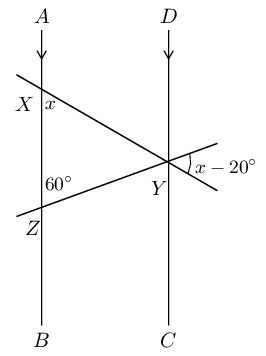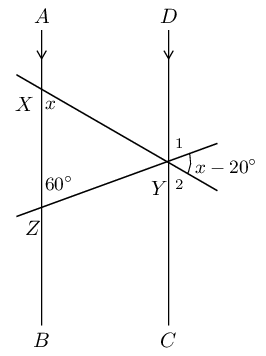$$\hat{Y}_{1} = 60°$$ (corresp $$\angle$$s; $$AB \parallel DC$$).

$$\hat{Y}_{2} = x$$ (corresp $$\angle$$s; $$AB \parallel DC$$).

\begin{align*} \therefore x + 60° + (x - 20°) &= 180° \qquad \text{(}\angle \text{s on a str line)}\\ 2x &= 180° - 40° \\ 2x &= 140° \\ \therefore x &= 70° \end{align*}

Find each of the unknown angles marked in the figure below. Find a reason that leads to the answer in a single step.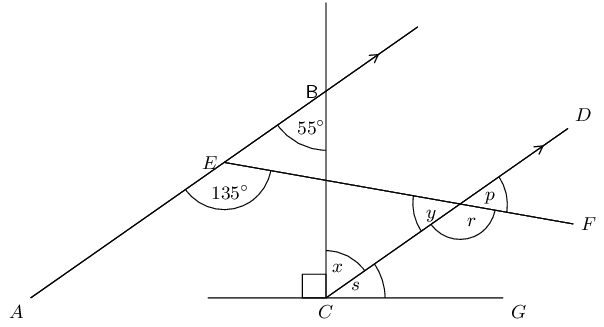$$\hat{x}$$

$$\hat{x}$$ and $$A\hat{B}C$$ are alternate interior angles on transversal $$BC$$. Therefore, they must be equal in size since $$AB \parallel CD$$.

Therefore $$\hat{x} = 55^{\circ}$$.

$$\hat{s}$$

We have just found that $$\hat{x} = 55^{\circ}$$. $$\hat{x} + \hat{s} + 90^{\circ} = 180^{\circ}$$ ($$\angle$$s on a str line)

\begin{align*} \hat{s} & = 90^{\circ} - 55^{\circ} \\ & = 35^{\circ} \end{align*}
$$\hat{r}$$

$$\angle A\hat{E}F$$ and $$\hat{r}$$ are corresponding angles ($$AB \parallel CD$$).

Therefore: $$\hat{r} = 135^{\circ}$$.

$$\hat{y}$$

$$\hat{r} + \hat{y} = 180^{\circ}$$ ($$\angle$$s on a str line).

\begin{align*} \hat{y} & = 180^{\circ} - 135^{\circ} \\ & = 45^{\circ} \end{align*}
$$\hat{p}$$

$$\hat{p} = \hat{y}$$ (vert opp $$\angle$$s $$=$$)

Therefore: $$\hat{p} = 45^{\circ}$$.

Based on the results for the angles above, is $$EF \parallel CG$$?

To prove $$EF \parallel CG$$ we need to show that one of the following is true:

• $$\hat{s} = \hat{p}$$ (corresp $$\angle$$s)
• $$\hat{s} = \hat{y}$$ (alt $$\angle$$s)
• $$\hat{s} + \hat{r} = 180^{\circ}$$ (co-int $$\angle$$s)

However $$\hat{s} \ne \hat{p}$$, therefore $$EF$$ is not parallel to $$CG$$.

Find each of the unknown angles marked in the figure below. Find a reason that leads to the answer in a single step.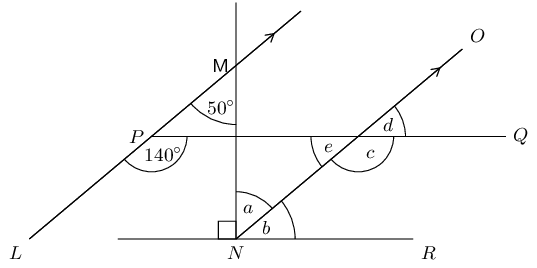$$\hat{a}$$

$$\hat{a}$$ and $$L\hat{M}N$$ are alternate interior angles on transversal $$MN$$. Since $$LM \parallel NO$$ they must be equal in size.

Therefore $$\hat{a} = 50^{\circ}$$.

$$\hat{b}$$

We have just found that $$\hat{a} = 50^{\circ}$$. $$\hat{a} + \hat{b} + 90^{\circ} = 180^{\circ}$$ ($$\angle$$s on a str line)

\begin{align*} \hat{b} & = 90^{\circ} - 50^{\circ} \\ & = 40^{\circ} \end{align*}
$$\hat{c}$$

$$\angle L\hat{P}Q$$ and $$\hat{c}$$ are corresponding angles ($$LM \parallel NO$$).

Therefore: $$\hat{c} = 140^{\circ}$$.

$$\hat{e}$$

$$\hat{c} + \hat{e} = 180^{\circ}$$ ($$\angle$$s on a str line).

\begin{align*} \hat{e} & = 180^{\circ} - 140^{\circ} \\ & = 40^{\circ} \end{align*}
$$\hat{d}$$

$$\hat{d} = \hat{e}$$ (vert opp $$\angle$$s $$=$$).

Therefore: $$\hat{d} = 40^{\circ}$$.

Based on the results for the angles above, is $$PQ \parallel NR$$?

To prove $$PQ \parallel NR$$ we need to show that one of the following is true:

• $$\hat{b} = \hat{d}$$ (corresp $$\angle$$s)
• $$\hat{b} = \hat{e}$$ (alt $$\angle$$s)
• $$\hat{b} + \hat{c} = 180^{\circ}$$ (co-int $$\angle$$s)

$$\hat{b} = \hat{d}$$ (corresp $$\angle$$s), therefore $$PQ \parallel NR$$. We also note that $$\hat{b} = \hat{e}$$ and $$\hat{b} + \hat{c} = 180^{\circ}$$.

Determine whether the pairs of lines in the following figures are parallel: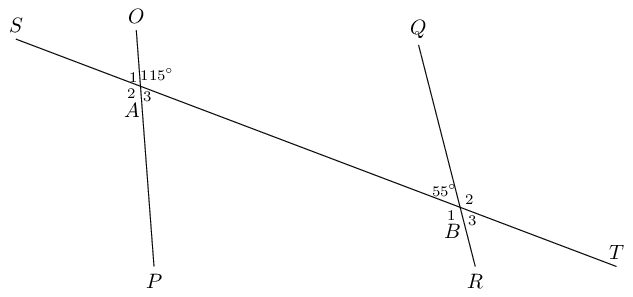If $$OP \parallel QR$$ then $$O\hat{A}B + Q\hat{B}A = 180°$$ (co-int $$\angle$$s). But $$O\hat{A}B + Q\hat{B}A = 115° + 55° = 170°$$. Therefore there are no parallel lines, $$OP$$ is not parallel to $$QR$$. Note that we do not consider $$ST$$ as this is a transversal.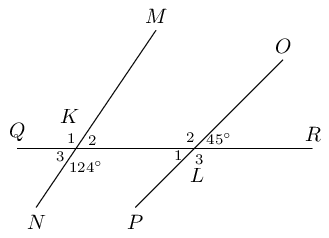$$K_2 = 180° - 124° = 56°$$ ($$\angle$$s on a str line). If $$MN \parallel OP$$ then $$\hat{K}_2$$ would be equal to $$\hat{L}$$, $$\therefore MN$$ is not parallel to $$OP$$. Note that $$QR$$ is a transversal.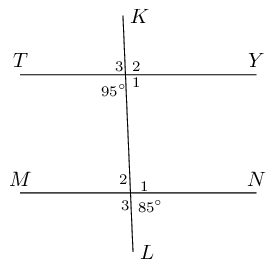Let $$U$$ be point of intersection of lines $$KL$$ and $$TY$$ and $$V$$ be the point of intersection of lines $$KL$$ and $$MN$$.

\begin{align*} \hat{U}_4 &= 95° \\ \hat{U}_1 &= 180° - 95° \qquad \text{(} \angle \text{s on a str line)}\\ \hat{U}_1 &= 85° \\ \hat{V}_4 &= 85° \qquad \text{(given)} \\ \therefore \hat{V}_{4} & = \hat{U}_1 \end{align*}

These are corresponding angles $$\therefore TY \parallel MN$$.

If $$AB$$ is parallel to $$CD$$ and $$AB$$ is parallel to $$EF$$, explain why $$CD$$ must be parallel to $$EF$$.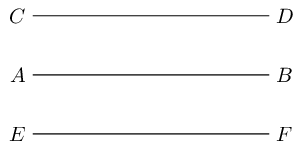If $$a = 2$$ and $$b = a$$ then we know that $$b = 2$$.

Similarly if $$AB \parallel CD$$ and $$EF \parallel AB$$ then we know that $$EF \parallel CD$$.